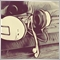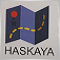# I'm trying to subtract my profits and add my losses, would this work?189

I'm trying to write a code that will make a "Profit Target" which will subtract my profits in the past 24 hours and add my losses. But I think there is something wrong, can anyone help?

```int ProfitTarget = 1000

{
for(int q = 0; q<OrdersHistoryTotal(); q++)
{
OrderSelect(q,SELECT_BY_POS,MODE_HISTORY);
if(OrderCloseTime()>TimeCurrent()-86400)
{
ProfitTarget+OrderProfit();
}
}
}```1780

```int ProfitTarget = 1000;
double TotalProfit=0;

{
for(int q = 0; q<OrdersHistoryTotal(); q++)
{
OrderSelect(q,SELECT_BY_POS,MODE_HISTORY);
if(OrderCloseTime()>TimeCurrent()-86400)
{
TotalProfit=TotalProfit+OrderProfit();
}
}
Print(" Daily Profit: "+ProfitTarget-TotalProfit);
}```